How Cheenta works to ensure student success?
Explore the Back-Story

# RMO 2010 | Regional Mathematics Olympiad ProblemsIn this post, there are problems from Regional Mathematics Olympiad, RMO 2010. Try out these problems.

1. Let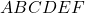be a convex hexagon in which the diagonals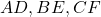are concurrent at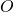. Suppose the area of triangle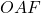is the geometric mean of those of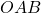and; and the area of the triangle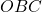is the geometric mean of those ofand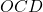. Prove that the area of triangle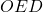is the geometric mean of those ofand.
2. Let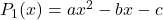,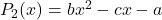,be three quadratic polynomials where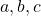are non-zero real numbers. Suppose there exits a real number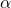such that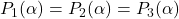. Prove that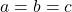.
3. Find the number of-digit numbers (in base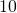) having non-zero digits and which are divisible bybut not by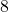.
4. Find three distinct positive integers with the least possible sum such that the sum of the reciprocals of any two integers among them is an integral multiple of the reciprocal of the third integer.
5. Let ABC be a triangle in which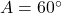Letand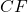be the bisectors of the angles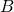and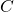with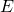on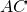and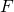on. Letbe the reflection ofin the line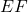. Prove thatlies on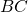.
6. For each integer 1 ≤ n, define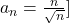where [x] denotes the largest integer not exceeding x, for any real numbers x. Find the number of all n in the set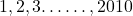for which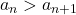.

RMO 2002 Problem 2 – Fermat’s Last Theorem as a guessing tool– Video

In this post, there are problems from Regional Mathematics Olympiad, RMO 2010. Try out these problems.

1. Letbe a convex hexagon in which the diagonalsare concurrent at. Suppose the area of triangleis the geometric mean of those ofand; and the area of the triangleis the geometric mean of those ofand. Prove that the area of triangleis the geometric mean of those ofand.
2. Let,,be three quadratic polynomials whereare non-zero real numbers. Suppose there exits a real numbersuch that. Prove that.
3. Find the number of-digit numbers (in base) having non-zero digits and which are divisible bybut not by.
4. Find three distinct positive integers with the least possible sum such that the sum of the reciprocals of any two integers among them is an integral multiple of the reciprocal of the third integer.
5. Let ABC be a triangle in whichLetandbe the bisectors of the anglesandwithonandon. Letbe the reflection ofin the line. Prove thatlies on.
6. For each integer 1 ≤ n, definewhere [x] denotes the largest integer not exceeding x, for any real numbers x. Find the number of all n in the setfor which.

RMO 2002 Problem 2 – Fermat’s Last Theorem as a guessing tool– Video

This site uses Akismet to reduce spam. Learn how your comment data is processed.

### Knowledge Partner